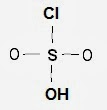Electron Lewis Dot Structure of chlorosulphonic acid HSO3Cl | Chemistry Net

# Electron Lewis Dot Structure of chlorosulphonic acid HSO3Cl

A simple procedure for writing electron Lewis dot structures was given in a previous post entitled “Lewis Structures and the Octet Rule”.
Several worked examples relevant to this procedure were given in previous posts please see the Sitemap - Table of Contents (Lewis Electron Dot Structures).

Let us examine the case of chlorosulphonic acid HSO3Cl . Chlorosulphonic acid is used to prepare alkyl sulfates, which are useful as detergents and as chemical intermediates. How can we construct the chlorosulphonic acid Lewis dot structure?

Step 1: Connect the atoms with single bonds.Fig. 1 : Connect the atoms of chlorosulphonic acid with single bonds.

Step 2:  Calculate the # of electrons in π bonds (pi bonds, multiple bonds) using  formula (1)

Where n in this case is 5 since  HSO3Cl  consists of six atoms but one of them is a H atom.
Where V = (1 + 6 + 6 * 3 + 7) = 26
Therefore, P = 6n + 2 – V = 6 * 5 + 2 – 32 = 0     So  there are no  π electrons (pi electrons) in HSO3Cl

Step 3 & 4: The Lewis structures of  HSO3Cl are derived below:Fig. 2 : Plausible Lewis structure of the chlorosulphonic acid.

#### 1 comment:

1.Alfa Chemistry employs more than 200 full time staff, of which approximate 80 are Ph.D. and M.S. chemists, specialized in synthetic chemistry, process optimization, and research. Acid Red 57